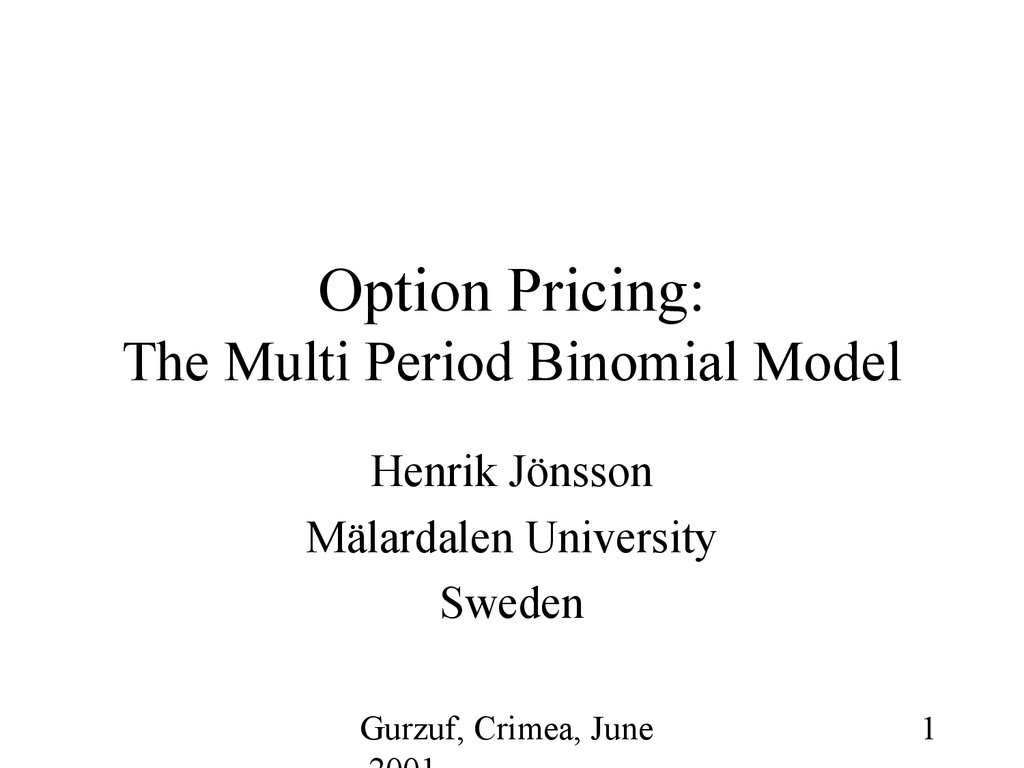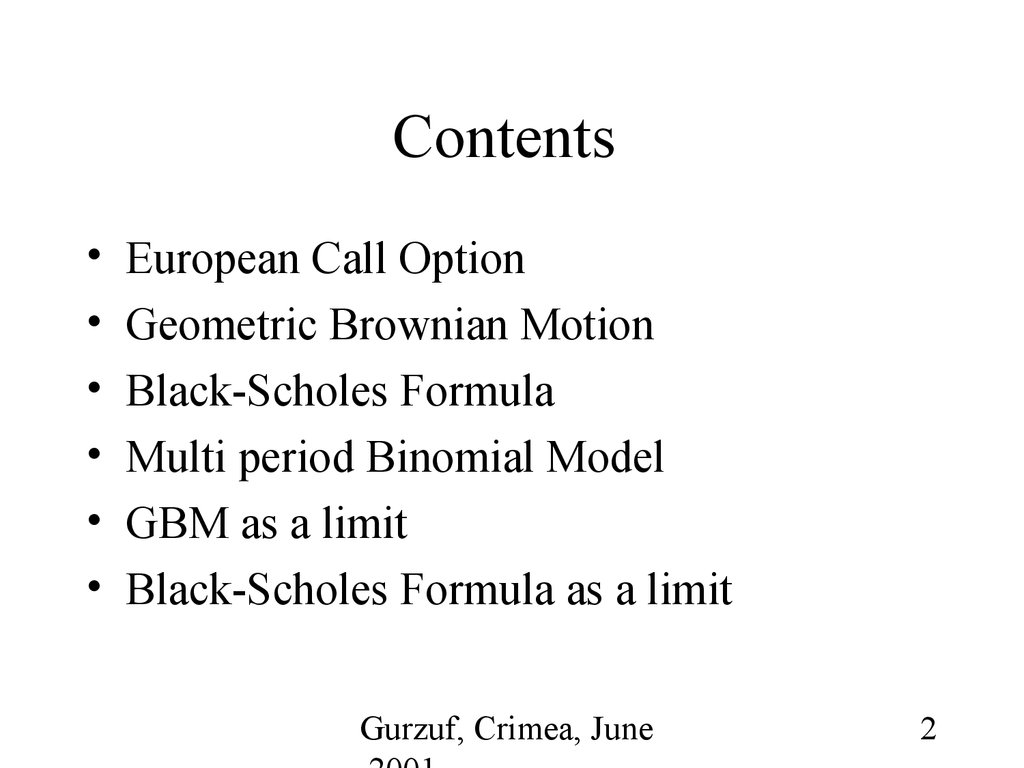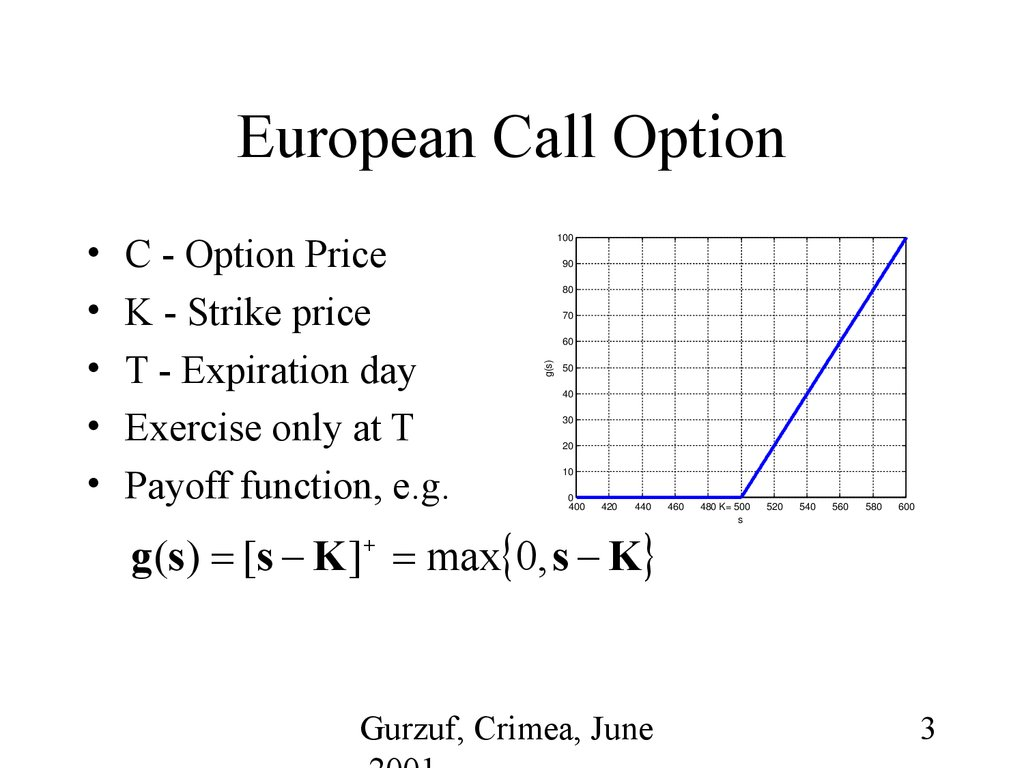# The binomial model for option pricing

## 1. Option Pricing: The Multi Period Binomial Model

Henrik Jönsson
Mälardalen University
Sweden
Gurzuf, Crimea, June
1

## 2. Contents

European Call Option
Geometric Brownian Motion
Black-Scholes Formula
Multi period Binomial Model
GBM as a limit
Black-Scholes Formula as a limit
Gurzuf, Crimea, June
2

## 3. European Call Option

C - Option Price
K - Strike price
T - Expiration day
Exercise only at T
Payoff function, e.g.
90
80
70
60
g(s)
100
50
40
30
20
10
0
400
420
440
g (s) [s K ] max 0, s K
460
480 K= 500
s
520
540
560
580
600
Gurzuf, Crimea, June
3

## 4. Geometric Brownian Motion

S(y), 0 y<t, follows a geometric Brownian
motion if
S (t y )
S(y )
independent of all prices up to time y
S (t y )
2
ln
~
N
t
,
t

S( y )
Gurzuf, Crimea, June
4

## 5. Black-Scholes Formula

The price at time zero of a European call
option (non-dividend-paying stock):
C S(0) ( ) Ke ( t )
rt
where
2
rt t ln K S(0)
2
t
Gurzuf, Crimea, June
5

## 6. The Multi Period Binomial Model

S
u 3S 0
u 2S 0
uS 0
S0
2
u dS 0
pi
uS i 1
Si
prob
1 pi
dS i 1
udS 0
i=1,2,…
dS 0
2
ud S 0
Note:
d 2S 0
3
d S0
i
Gurzuf, Crimea, June
• u and d the same for all
moments i
• d < 1+r < u, where r is
the risk-free interest rate
6

## 7. The Multi Period Binomial Model

• Let
1 if S i uS i 1
Xi
0 if S i dS i 1
i=1,2,…
• Let (X1, X2,…, Xn) be the vector describing
the outcome after n steps.
• Find the set of probabilities
P{X1=x1, X2 =x2,…, Xn =xn},
xi=0,1, i=1,…,n,
such that there is no arbitrage opportunity.
Gurzuf, Crimea, June
7

## 8. The Multi Period Binomial Model

• Choose an arbitrary vector ( 1, 2, …, n-1)
• If A={X1= 1, X2= 2, …, Xn-1= n-1} is true
buy one unit of stock and sell it back at
moment n
• Probability that the stock is purchased
qn-1=P{X1= 1, X2= 2, …, Xn-1= n-1}
• Probability that the stock goes up
pn= P{Xn=1| X1= 1, …, Xn-1= n-1}
Gurzuf, Crimea, June
8

## 9. The Multi Period Binomial Model

S
u 3S 0
pi
uS i 1
Si
prob
dS
1 pi
i 1
u 2S 0
uS 0
S0
u 2 dS 0
udS 0
dS 0
Example:
ud 2 S 0
(0,1,1)
q 3 P{X1 0, X 2 1, X 3 1}
d 2S 0
p 4 P{X 4 1 X1 0, X 2 1, X 3 1}
3
d S0
1
2
i
Gurzuf, Crimea, June
3
n=4
9

## 10. The Multi Period Binomial Model

• Expected gain =
qn-1[pn(1+r)-1uSn-1+(1- pn) (1+r)-1dSn-1-Sn-1]
r = risk-free interest rate
• No arbitrage opportunity implies
1 r d
pn
u d
Gurzuf, Crimea, June
10

## 11. The Multi Period Binomial Model

• ( 1, 2, …, n-1) arbitrary vector
• No arbitrage opportunity
X1,…, Xn independent with
P{Xi=1}=p, i=1,…,n
1 r d
p
u d
Risk-free interest rate r the
same for all moments i
Gurzuf, Crimea, June
11

## 12. The Multi Period Binomial Model

Limitations:
• Two outcomes only
• The same increase &
decrease for all time
periods
• The same probabilities
Qualities:
• Simple mathematics
• Arbitrage pricing
• Easy to implement
Gurzuf, Crimea, June
12

## 13. Geometric Brownian Motion as a Limit

The Binomial process:
p
t
t u
S( j ) S(( j 1) ) prob
,
1 p
n
n d
t
n
rt
1
u e
n d
p
t ,
u
d
n
d e
and
j 1, 2,..., n
rt
one period risk free interest rate
n
Gurzuf, Crimea, June
13

## 14.

The Binomial Process
S
u 3S 0
u 2S 0
uS 0
u 2 dS 0
S0
udS 0
dS 0
ud 2 S 0
d 2S 0
d 3S 0
t
n
2t
n
3t
n
Gurzuf, Crimea, June
t
i
14

## 15. GBM as a limit

Let
t
t
1 if S( j n ) uS (( j 1) n )
Xj
t
t
0 if S( j ) dS (( j 1) )
n
n
n
and Y X j , Y ~ Bin(n,p)
j 1
Gurzuf, Crimea, June
15

## 16. GBM as a Limit

The stock price after n periods
S(t ) S(0)u Y d n Y
Y
u
S(0) d n
d
2
S(0)e
S(0)e W
where
W 2
t
Y
n
e
nt
t
Y nt
n
Gurzuf, Crimea, June
16

## 17. GBM as a Limit

Taylor expansion
t
n
t
2 t
u e
1
n
2n
t
t
2 t
n
d e
1
n
2n
gives
1 rtn d 1 r nt nt
p
u d
2 2
4
Gurzuf, Crimea, June
17

## 18. GBM as a limit

Expected value of W
t
EY nt
n
1
2 nt (p )
2
r nt nt
2 nt (
)
2
4
2
(r )t
2
EW 2
Variance of W
2
VarW
t
2 VarY
n
t
4 2 np (1 p)
n
2t
EY = np
VarY = np(1-p)
Gurzuf, Crimea, June
18

## 19. GBM as a limit

S(t ) S(0)e W ,
w 2
t
Y nt
n
By Central Limit Theorem
S (t )
2
2
W ln
~ N (r )t, t as n
2
S ( 0)
Gurzuf, Crimea, June
19

## 20. GBM as a limit

The multi period Binomial model becomes
geometric Brownian motion when n → ∞,
since
t
S j
n
, j 1,..., n,
t
S ( j 1)
n
are independent
2
S (t )
2
ln
~ N (r )t, t
2
S(0)
Gurzuf, Crimea, June
20

## 21. B-S Formula as a limit

n
• Let Y X i , Y ~ Bin(n,p)
i 1
• The value of the option after n periods =
max[S(t)-K,0] = [S(t)-K]+
where S(t)= uY dn-Y S(0)
• No arbitrage
C (1
rt - n
) E[S(t) - K]
n
Gurzuf, Crimea, June
21

## 22. B-S formula as a limit

The unique non-arbitrage option price
Y
rt
u n
C 1 E S(0) d K
n
d
n
rt
1 E S(0)e W K
n
n
As n → ∞
C e E S(0)e
- rt
2
( r ) t tX
2
w 2
t
n
Y nt
K ,
Gurzuf, Crimea, June
X~N(0,1)
22

## 23. B-S formula as a limit

C e E S(0)e
- rt
e S(0)e
A
- rt
2
( r ) t tX
2
2
( r ) t tx
2
where X~N(0,1) and A
K
K f X (x)dx
1 2
K
t rt ln
x : x
t 2
S(0)
Gurzuf, Crimea, June
23

## 24. B-S formula as a limit

e S(0)e
A
- rt
e - rt S(0)e
2
( r ) t tx
2
(r
2
) t tx
2
A
K f X (x)dx
f X (x)dx - e - rt K f X (x)dx
A
S(0)I 1 e rt KI 2
Gurzuf, Crimea, June
24

## 25. B-S formula as a limit

I1
A
B
1
2
1
2
e
e
2
x2
t tx
2
2
y2
2
dx
dx where A {x : x t ( t rt ln SK( 0 ) )}
2
2
1
where y x t and
B {y : y 1 t ( 2 t rt ln SK( 0 ) t )}
where 1 t (rt 2 t ln SK( 0 ) )
2
( )
2
(·) is the N(0,1) distribution
function
Gurzuf, Crimea, June
25

## 26. B-S formula as a limit

I2
A
1
2
e
x2
2
dx
where A {x : x 1 t ( 2 t rt ln SK( 0 ) )}
2
( t ) where t (rt t ln SK( 0 ) )
1
Gurzuf, Crimea, June
2
2
26

## 27. B-S formula as a limit

C S(0) ( ) e rt K ( t ) as n
where
2
rt t ln K S(0)
2
t
Gurzuf, Crimea, June
27